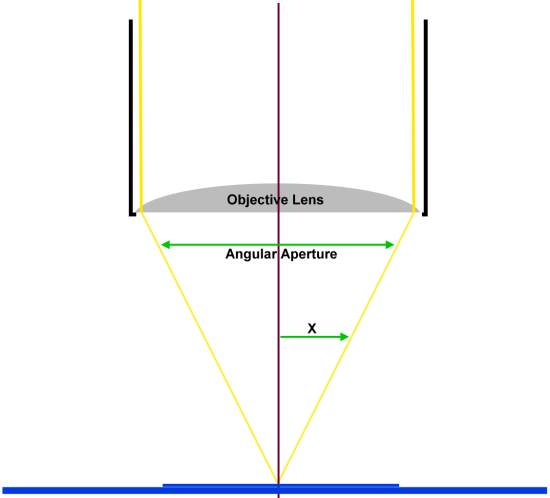# Numerical Aperture

Numerical Aperture is a number etched into the side of your objective lens that tells you how well the objective will resolve details.  It relates the diameter of the lens to the focal distance.  A wider lens will have a higher NA, and a lens that focuses closer to the subject will also have a higher NA.In the diagram above, the angle that the light makes is called the Angular Aperture. The Numerical Aperture is the sine of one half of that angle (labeled X in the diagram), multiplied by the refractive index of the medium between the slide and the lens.  For air, the refractive index is 1.0.  For immersion oil, it is closer to 1.51.

Oil immersion objective lenses are designed to have a drop of immersion oil between the slide and the objective lens.  This allows the lens to capture more of the light, and improves the resolution.

The maximum value that X can have is 90 degrees (since twice that is 180 degrees, which is a straight line, and would mean the lens was touching the slide).  The sine of 90 degrees is 1.0, which multiplied by the refractive index of air is still 1.0, so that is why the maximum NA of a dry objective is 1.0.  In practice, we can't actually squash the subject under the lens completely, and the angle is just under 90 degrees, giving a maximum NA of about 0.95.